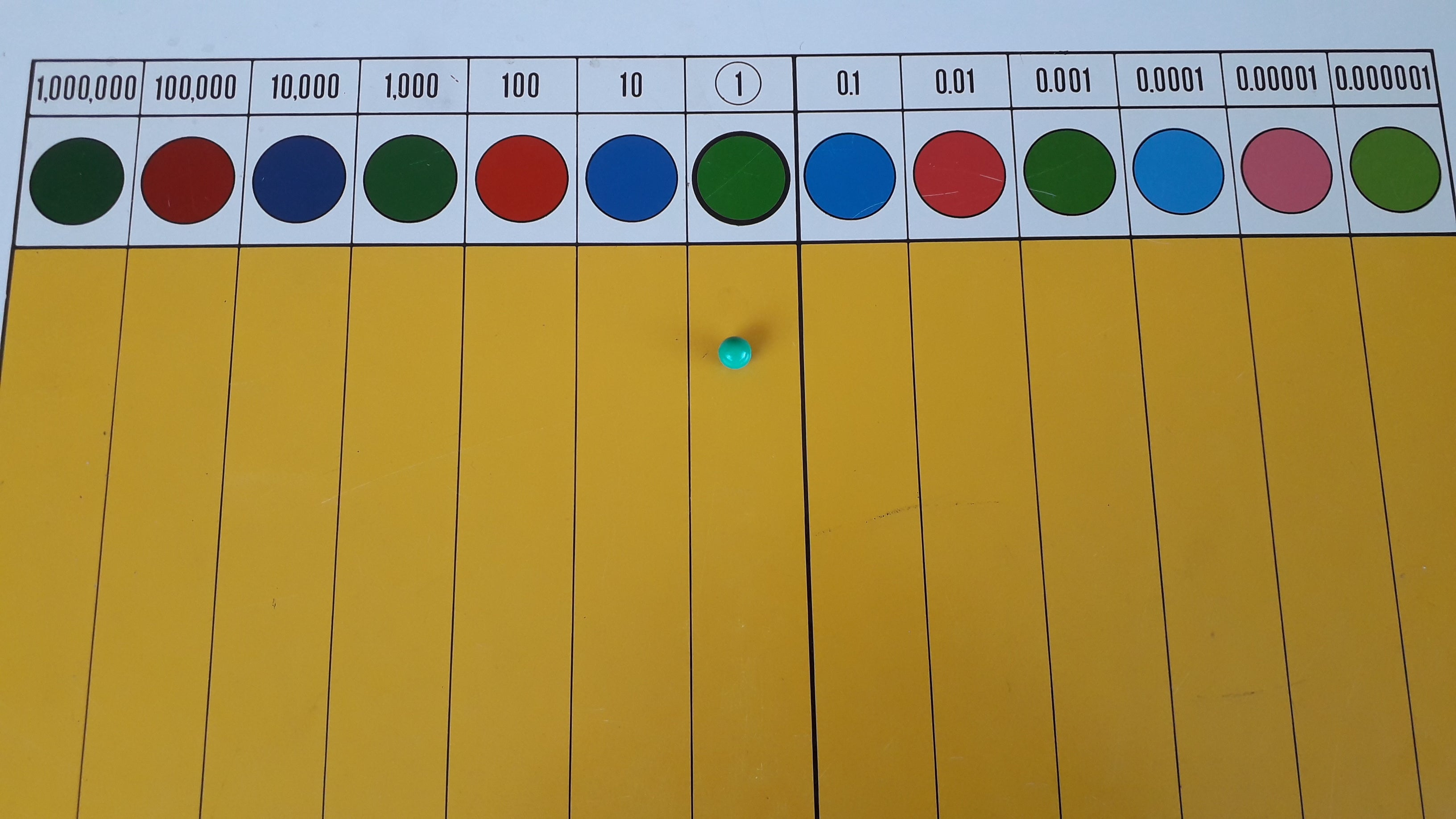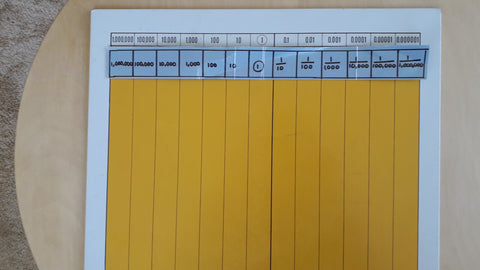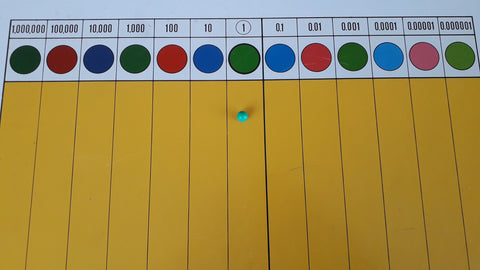# Introduction to the Montessori Decimal Board Part 1

Decimals can be hard for students to understand. Unlike whole number some students do not have a lot of exposure to these types of numbers. Importantly though they link to fractions and percentages and knowledge of them is vital for advanced maths.

In Montessori we have the Decimal Board to help introduce this concept.

For the second article in this series click here

The lesson - Introduction 1

Prior to this lesson students will need to

• Have a very secure knowledge of fractions
• Have a secure knowledge of ten e.g. ten units make ten, ten tens make one hundred

You will need to have a premade fraction strip to place on top of the decimal board.1) Place a green bead, golden bead and red circle inset (fraction circle) ask what these all are (1).

2) We are going to work with this green bead and pretend we are going to cut into 10. Why 10? (have students answer)

3) Show the blue disks and say these are each one tenth - point to the fraction strip at the top to show this

4) Take one tenth. Ask what it would be if I cut into ten bits (100ths) show the red/dark pink disk and say how these equal 100ths - point to the fraction strip at the top to show this

5) Continue across dark green: thousandths, light blue: ten ten-thousandths, light pink: ten hundred-thousandths, light green: ten millionths.

The lesson - Introduction 2

1) Produce a red bead. In the stamp game/checkerboard what is red? (hundreds) .

2) If I exchanged it what would I exchange it for? (ten blue beads - tens) . Ask a student to make this exchange.

3) Repeat with the blue beads for the ten green unit beads. Have a student exchange them.

4) Continue going into the 'decimals' make sure students know that 'th'goes at the end of the decimal words. It may be useful to over accentuate this sound so students hear it.

5) Students can ask a partner to 'exchange' a number for a number.

The lesson - Introduction 3

1) There is a number that we use a lot in maths. That number is the unit. Show the golden bead unit/ red whole fraction/ green bead.

2) We are going to use the green bead to show units today. We have been using this green bead when we use the decimal board.

3) Place the green unit bead in the units column4) Ask students what it stands for (1)

5) What is the number immediately to the left of this unit bead? (10)

6) What is the number immediately to the right of this unit bead (a tenth)

7) What do you notice about the numbers on either side of this unit? Put one finger in the tens and another finger in the tenths. (They both have the word ten in it)

8) Now point to the hundreds and the hundredths. What do you notice about these numbers? (They both have the word hundredths in them)

9) If possible place a laminated sheet or similar over the top of the decimal board and draw with curved lines the connections between the numbers e.g. draw a curved line that connects the tens to the tenths, another line that connects the hundreds to the hundredths, another line that connects the thousands to the thousandths and so on....

10) Conduct a three period lesson for the names of the numbers.

To see what comes next go to the second article in this series here